1. Worksheets>
2. Math>
3. Geometry>
4. Circle>
5. Circumference and Area

Circumference and Area of Circle Worksheets

This page contains worksheets in finding area and circumference of a circle with all possible combinations. There are three levels Easy, Moderate and Hard. Easy worksheets required exact answers with radius ranging from 1 to 25. Moderate worksheets required rounding answers to tenth place value with radius ranging more than 25 but less 100. Hard worksheets contain the radius in decimals and required answers rounded to two decimal places. Use pi = 3.14 wherever required.

Select the Measurement Units

Applying the formula, Area = pi * r2, find the area of each circle.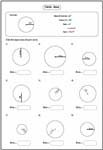Easy:

Moderate:

Difficult:

Area from Diameter

Find the radius from diameter and apply the formula to find the area of a circle.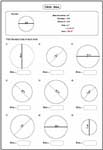Easy:

Moderate:

Difficult:

Area from Radius or Diameter

Each worksheet has 9 problems finding area of a circle with the known radius or diameter. Two simple word problems and one story problem included.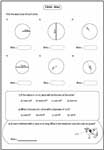Easy:

Moderate:

Difficult:

Area and Circumference: Combined Review

These are the perfect review worksheets in finding both area and circumference of the circle.Easy:

Moderate:

Difficult:

Radius or Diameter from Area

Dividing the area by pi or 3.14, you get r2. Taking the square root, you get radius. Solve each problem based on this technique.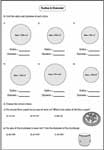Easy:

Difficult:

Area from Circumference

Find the area from the circumference of each circle. Word problems included to understand real-life application.Easy:

Moderate:

Difficult:

Area between Concentric Circles (or ring)

Find the area of outer circle and inner circle. Subtract the inner area from the outer area to find the area of the ring.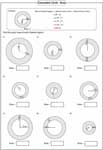Easy:

Moderate:

Difficult:

Applying the formula, Circumference = 2 * pi * r, find the circumference of each circle.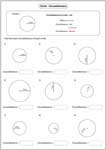Easy:

Moderate:

Difficult:

Circumference from Diameter

To find the circumference, multiply diameter with pi value. Each worksheet has 9 problems.Moderate:

Difficult:

Circumference from Radius or Diameter

Circumference of a circle worksheet contains six standard problems and two word problems.Easy:

Moderate:

Difficult:

Radius or Diameter from Circumference

Divide the circumference by pi or 3.14. You get the diameter. Answer the questions.Easy:

Difficult:

Circumference from Area

Each worksheet has 8 problems finding circumference from area of a circle. Simple word problems included.Easy:

Moderate:

Difficult: